# General solution for high dynamic range three-dimensional shape measurement using the fringe project

135

General solution for high dynamic range three-dimensional shape measurement using the fringe projection technique

Shijie Feng, Yuzhen Zhang, Qian Chen, Chao Zuo, Rubin Li and Guochen Shen

Jiangsu Key Laboratory of Spectral Imaging & Intelligent Sense,

Nanjing University of Science and Technology, Nanjing, Jiangsu Province 210094, China

Abstract

This paper presents a general solution for realizing high dynamic range three-dimensional (3-D) shape measurement based on fringe projection. Three concrete techniques are involved in the solution for measuring object with large range of reflectivity (LRR) or one with shiny specular surface. For the first technique, the measured surface reflectivities are sub-divided into several groups based on its histogram distribution, then the optimal exposure time for each group can be predicted adaptively so that the bright as well as dark areas on the measured surface are able to be handled without any compromise. Phase-shifted images are then captured at the calculated exposure times and a composite phase-shifted image is generated by extracting the optimally exposed pixels in the raw fringes images. For the second technique, it is proposed by introducing two orthogonal polarizers which are placed separately in front of the camera and projector into the first technique and the third one is developed by combining the second technique with the strategy of properly altering the angle between the transmission axes of the two polarizers. Experimental results show that the first technique can effectively improve the measurement accuracy of diffuse objects with LRR, the second one is capable of measuring object with weak specular reflection (WSR: e.g. shiny plastic surface) and the third can inspect surface with strong specular reflection (SSR: e.g. highlight on aluminum alloy) precisely. Further, more complex scene, such as the one with LRR and WSR, or even the one simultaneously involving LRR, WSR and SSR, can be measured accurately by the proposed solution.

Files

PDF

Citations

Feng, S., Zhang, Y., Chen, Q., Zuo, C., Li, R., & Shen, G. (2014). General solution for high dynamic range three-dimensional shape measurement using the fringe projection technique. Optics and Lasers in Engineering, 59, 56-71.

Results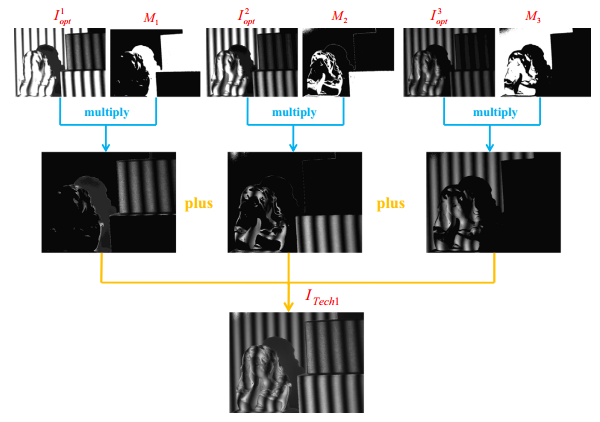Fig. 1. Fringe image fusion process of the Technique I.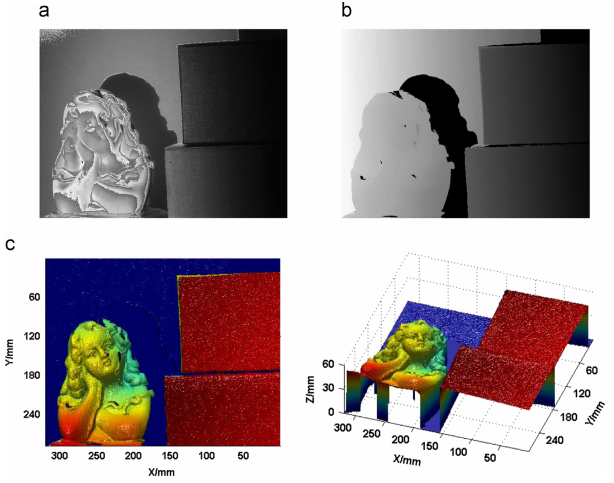Fig. 2. Resultant images of the Technique I. (a) A formed low frequency fringe image by the Technique I; (b) calculated absolute phase map; and (c) 3-D results in different views.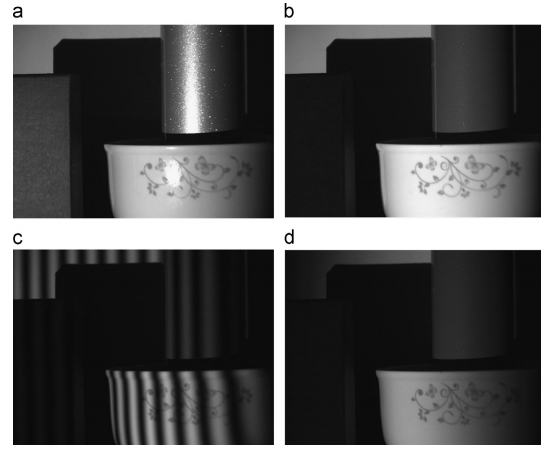Fig. 3. Effect of WSR and experimental images of the traditional method. (a) Measured scene without highlight elimination; (b) the scene after introducing the two perpendicular polarizers (image I0); (c) representative fringe image of high frequency; and (d) representative fringe image of low frequency.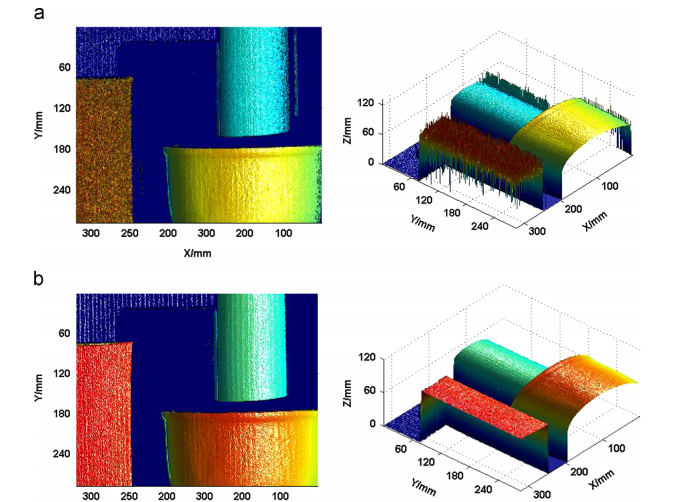Fig. 4. 3-D results by the two methods. (a) 3-D reconstructed models by traditional method; and (b) 3-D reconstructed models by the Technique II.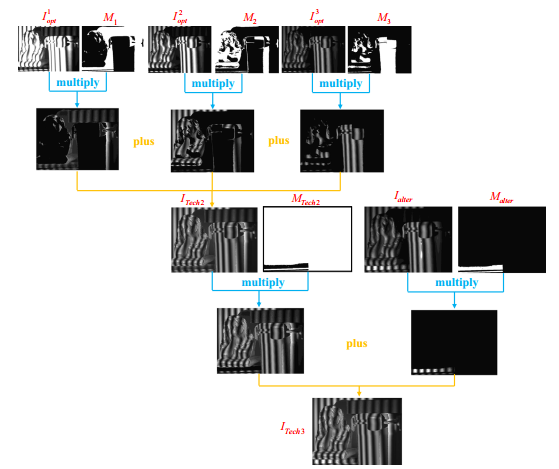Fig. 5. .Fringe image fusion process of the Technique III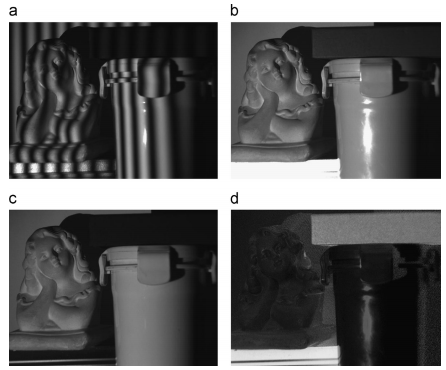Fig. 6. Images about angle alteration. (a) Captured fringe image after the angle adjustment of 41; (b) image Imax captured when the angle θ being 01; (c) image Imax captured when the angle θ being 901; and (d) calculated map of degree of polarization.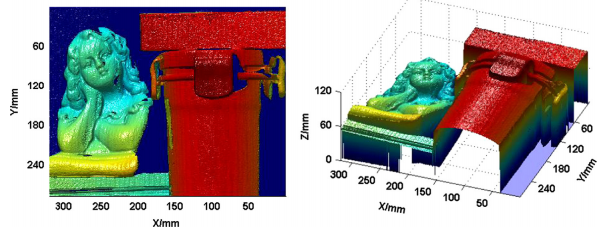Fig. 7. 3-D reconstruction results of the Technique III.

Methods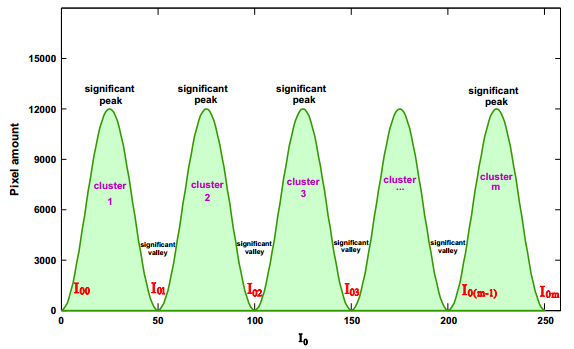Fig. 1. Example of histogram of .

The reflectivity α and the captured image are linear, which means that the variation of can be directly used to represent the variation of reflectivity. To obtain the distribution of , we calculate the histogram of I0, in which the horizontal axis shows the variation of intensity of and vertical one depicts the amount of pixels in that particular . An example of histogram is shown in Fig. 1. The region of light green represents the whole variation range of the captured intensity . By the proposed method, the whole large variation range is further divided into several clusters composed of pixels varying in small range of . Since the pixels within a certain cluster have similar reflectivity, a single exposure would be sufficient for a single cluster.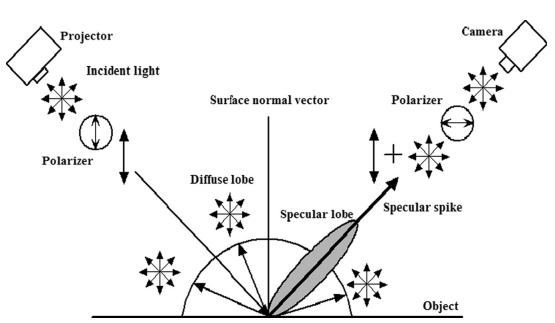Fig. 2. Schematic diagram of the Technique II.

To eliminate the effect of specular highlight, polarization method is introduced and Fig. 2 demonstrates its schematic diagram. Two polarized filters are placed in front of the projector and camera respectively. The projected incident light turns into linear polarized light after it passes through the first polarizer and after the reflection occurs on measured object, the original linear polarized light has changed into a compound one composed of diffuse lobe, specular lobe and specular spike.

------------------------------------------------

Contact

Shijie Feng

Ph.D. Candidate of NJUST

Email:geniusshijie@163.com ( or 311040574@njust.edu.cn)

Nanjing University of Science and Technology, Jiangsu Province (210094), P.R.China

Qian Chen

Dean of the school of Electronic and Optical Engineering

Email: chenqian@njust.edu.cn

Nanjing University of Science and Technology, Jiangsu Province (210094), P.R.China

Chao Zuo

Associate professor at the school of Electronic and Optical Engineering

Email: surpasszuo@163.com

Nanjing University of Science and Technology, Jiangsu Province (210094), P.R.China